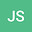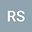Numerical solution of coupled Lane-Emden boundary value problems using the Bernstein collocation method
••• Julee Shahni,
• Randhir Singh
Julee Shahni
Birla Institute of Technology
Author ProfileRandhir Singh
Indian Institute of Technology Kharagpur
Author Profile## Abstract

In this paper, we provide an efficient numerical technique based on the Bernstein polynomials for numerical approximation of the coupled Lane-Emden type equation which arises in various fields of applied mathematics, physical and chemical sciences. We consider the equivalent integral form of the coupled Lane-Emden boundary value problems. The Bernstein collocation method is used to convert the integral equation into a system of nonlinear equations. This system is then solved efficiently by suitable iterative method. The error analysis of the current method is discussed. The accuracy of the proposed method is examined by calculating the maximum absolute error $L_{\infty}$, the $L_{2}$ error and the residual error of some numerical examples. The obtained numerical results are compared with the exact solutions and the results obtained by the other known techniques.